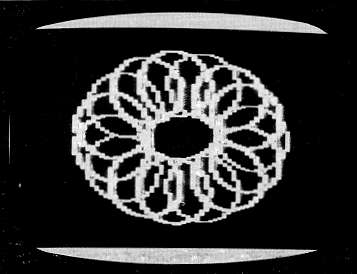`COMPUTE! ISSUE 37 / JUNE 1983 / PAGE 235`

 VIC Power Spirals Bruce Jordan Computer art in the form of "spiro-graphic" designs. Spiro is an excellent demonstration of the high resolution color graphics capability of the VIC 20 Superexpander module. You'll see almost anything -from geodesic domes to impossible bottles. "Spiro" works by choosing a screen location based on a value of a circular function; then, it uses the CIRCLE command of the Superexpander to draw a circle (I call them spiros) around the screen location. Next, the program loops back to the function, takes a new value, and the process is repeated until the design is complete.     When the program is run, it asks for information that gives you control over the final design. Creating Your Design The first thing that Spiro asks you is "How many spiros?" This determines how many times the spiro will be repeated across the screen. The higher the number, the more complex the design will be. Try 30 to start with.     Second, it asks "Alternate?" This allows you to create a design consisting of two different spiros. If you type Y, Spiro will ask you for the height and width of each of the two spiros to be used in your design. But if you type N, you will be asked for the height and width of a single spiro. Try N, and then 200 for the height and 150 for the width.     The last thing you are asked is which graphics mode you want your design to be drawn in. If you type 1, the design will be drawn in the multi color mode. If you choose 2, the design will be drawn in the high-resolution mode, and in black and white.     After the design is finished, you can return to the beginning of the program by typing R. This lets you alter your design by changing the parameters. If you type F after your design is complete, the program will automatically list the plotting function to allow for quick and easy editing.     Changing the plotting function will radically alter how your design will look. Depending on what function you use, you can wind up with anything from a geodesic sphere to a sort of improbable bottle that has its insides opening outwards to become its outsides! The best thing about Spiro is that it's unpredictable. Below is a list of some of the possible plotting functions. Try these, or make up your own and see what you get. Plotting Functions For Spiro 1. X=511+200*COS(A), Y=511+250*SIN(A) 2. X=511+200*COS(A), Y=511+250*SIN(A)T↑3 3. X=511+200*COS(A)↑3, Y=511+250*SIN(A)↑3*COS(A) 4. X=511+150*SIN(A)+200*COS(A), Y=511+325*SIN(A) 5. X=511+200*SIN(A)*COS(A), Y=511+250*SIN(A)One of the designs possible with a VIC-20 and "Power Spirals" 10 PRINT"{CLEAR}" 20 INPUT"HOW MANY SPIROS";Z 30 INPUT"ALTERNATE";A\$ 40 IFA\$="N"THEN90 50 INPUT"#1 HEIGHT";H1 60 INPUT"#1 WIDTH";W1 70 INPUT"#2 HEIGHT";H2 80 INPUT"#2 WIDTH";W2:GOTO110 90 INPUT"HEIGHT OF SPIRO";H1 100 INPUT"WIDTH OF SPIRO";W1 110 INPUT"GRAPHICS MODE";G 120 IFG=ITHENP=3:K=0 130 IFG=2THENP=0:K=1 140 GRAPHIC G:COLOR K,4,P,7 150 A=0:DA=2*Π/Z 160 I=1:DI=1 170 GOSUB 280 180 CIRCLE I,X,Y,W1,H1 190 IF A\$="Y" THEN GOSUB 350 200 A=A+DA:IF A=2*Π THEN 230 210 I=I+DI:IFI=>4THEN160 220 GOT0170 230 GETB\$:IFB\$=""THEN230 240 IFB\$="R"THEN260 250 IFB\$="F"THEN270 260 GRAPHIC 0:COLOR 1,2,0,4:GOTO 20 270 GRAPHIC 0:COLOR 1,2,0,4:LIST 280-290 280 X=511+200*COS(A) 290 Y=511+250*SIN(A) 300 IFX<=0THENX=0 310 IFX=>1023THENX=1023 320 IFY<=0THENY=0 330 IFY=>1023THENY=1023 340 RETURN 350 L=I:IFL<=2THENL=L+1:GOT0370 360 IFL=>3THENL=1 370 CIRCLE L,X,Y,W2,H2 380 RETURN